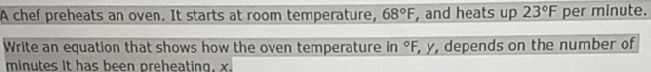### ¿Todavía tienes preguntas de matemáticas?

Pregunte a nuestros tutores expertos
Algebra
PreguntaA chef preheats an oven. It starts at room temperature, $$68 ^ { \circ } F$$ , and heats up $$23 ^ { \circ } F$$ per minute. Write an equation that shows how the oven temperature in $${ } ^ { \circ } F , y ,$$ depends on the number of minutes It has been preheating, $$x$$